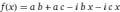# Is there a software that simplify mathematical expressions?

#### Michael George

Joined Feb 8, 2015
53
When I work with circuits that contains some capacitors, inductors and resistors and the supply is AC, I get long mathematical expressions and I have to do many calculations and many steps.
I would like to use an online website or a free software that is able to simplify these expressions. I need the software to support complex numbers or square root of (-1).

To clarify what I need, Here is a quick example:
I need the software to expand this expression (R1 - i * Xc) * (R2 + R3) to this expression
R1*R2 + R1*R3 - i*Xc*R2 - i*Xc*R3

Also, It is better if the software supports ratios.

I would be grateful if you give me a link or a software,
Thank you very much,

#### GopherT

Joined Nov 23, 2012
8,012
When I work with circuits that contains some capacitors, inductors and resistors and the supply is AC, I get long mathematical expressions and I have to do many calculations and many steps.
I would like to use an online website or a free software that is able to simplify these expressions. I need the software to support complex numbers or square root of (-1).

To clarify what I need, Here is a quick example:
I need the software to expand this expression (R1 - i * Xc) * (R2 + R3) to this expression
R1*R2 + R1*R3 - i*Xc*R2 - i*Xc*R3

Also, It is better if the software supports ratios.

I would be grateful if you give me a link or a software,
Thank you very much,

WolframAlpha.com

Will do it. You cannot have variables with two characters so change R1, R2 and R3 to a, b and c. Xc to x.

Just plug formula into search bar and it will solve for you.•MrSoftware and wayneh

#### anhnha

Joined Apr 19, 2012
886
When I work with circuits that contains some capacitors, inductors and resistors and the supply is AC, I get long mathematical expressions and I have to do many calculations and many steps.
I would like to use an online website or a free software that is able to simplify these expressions. I need the software to support complex numbers or square root of (-1).

To clarify what I need, Here is a quick example:
I need the software to expand this expression (R1 - i * Xc) * (R2 + R3) to this expression
R1*R2 + R1*R3 - i*Xc*R2 - i*Xc*R3

Also, It is better if the software supports ratios.

I would be grateful if you give me a link or a software,
Thank you very much,
These things can be done easily in Mathematica or Maple.

•Michael George

#### GopherT

Joined Nov 23, 2012
8,012
These things can be done easily in Mathematica or Maple.
Mathematica is made by Wolfram Research - same guy that offers Wolframalpha.com - for free (handle all this simple stuff).

Last edited:
•Michael George

#### MrSoftware

Joined Oct 29, 2013
2,015
Whoever put together the Wolfram Alpha web site was one bright individual. That site amazes me every time I use it.

•Michael George